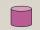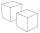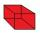# Quadratic equation + surface area - math problems

#### Number of problems found: 27

• Rectangle poolFind dimensions of an open pool with a square bottom with a capacity of 32 m3 to have painted/bricked walls with the least amount of material.
• Cuboid - ratiosThe sizes of the edges of the cuboid are in the ratio 2: 3: 5. The smallest wall has an area of 54 cm2. Calculate the surface area and volume of this cuboid.
• Hexagonal prism 2The regular hexagonal prism has a surface of 140 cm2 and height of 5 cm. Calculate its volume.
• Cylinder diameterThe surface of the cylinder is 149 cm2. The cylinder height is 6 cm. What is the diameter of this cylinder?
• Rectangular cuboidThe rectangular cuboid has a surface area 5334 cm2, and its dimensions are in the ratio 2:4:5. Find the volume of this rectangular cuboid.
• CubesSurfaces of cubes, one of which has an edge of 48 cm shorter than the other, differ by 36288 dm2. Determine the length of the edges of this cubes.
• Special cubeCalculate the edge of cube, if its surface and its volume is numerically equal number.

We apologize, but in this category are not a lot of examples.
Do you have an exciting math question or word problem that you can't solve? Ask a question or post a math problem, and we can try to solve it.

We will send a solution to your e-mail address. Solved examples are also published here. Please enter the e-mail correctly and check whether you don't have a full mailbox.

Looking for help with calculating roots of a quadratic equation? Quadratic Equations Problems. Examples for the calculation of the surface area of ​​the solid object .